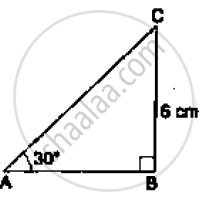# In the Adjoining Figure, δAbc is Right-angled at B and ∠A = 300. If Bc = 6cm, Find (I) Ab, (Ii) Ac. - Mathematics

In the adjoining figure, ΔABC is right-angled at B and ∠A = 300. If  BC = 6cm, find (i) AB, (ii) AC.

#### SolutionFrom the given right-angled triangle, we have:

(BC)/(AB)= tan 30^0

⇒6/(AB) = 1/sqrt(3)

⇒ AB = 6 sqrt(3) cm

Also, (BC)/(AC) = sin 30^0

⇒6/(AC)=1/2

⇒ AC = (2xx6)=12 cm

∴ AB = 6sqrt(3) cm and AC = 12 cm

Concept: Trigonometric Ratios and Its Reciprocal
Is there an error in this question or solution?
Chapter 6: T-Ratios of some particular angles - Exercises

#### APPEARS IN

RS Aggarwal Secondary School Class 10 Maths
Chapter 6 T-Ratios of some particular angles
Exercises | Q 21
Share